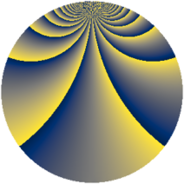# Properties

 Label 1045.2.ciLevel $1045$ Weight $2$ Character orbit 1045.ci Rep. character $\chi_{1045}(27,\cdot)$ Character field $\Q(\zeta_{60})$ Dimension $1856$ Sturm bound $240$

# Related objects

## Defining parameters

 Level: $$N$$ $$=$$ $$1045 = 5 \cdot 11 \cdot 19$$ Weight: $$k$$ $$=$$ $$2$$ Character orbit: $$[\chi]$$ $$=$$ 1045.ci (of order $$60$$ and degree $$16$$) Character conductor: $$\operatorname{cond}(\chi)$$ $$=$$ $$1045$$ Character field: $$\Q(\zeta_{60})$$ Sturm bound: $$240$$

## Dimensions

The following table gives the dimensions of various subspaces of $$M_{2}(1045, [\chi])$$.

Total New Old
Modular forms 1984 1984 0
Cusp forms 1856 1856 0
Eisenstein series 128 128 0

## Trace form

 $$1856 q - 18 q^{2} - 18 q^{3} - 2 q^{5} - 12 q^{6} - 36 q^{7} + O(q^{10})$$ $$1856 q - 18 q^{2} - 18 q^{3} - 2 q^{5} - 12 q^{6} - 36 q^{7} - 48 q^{10} - 72 q^{11} - 18 q^{13} - 18 q^{15} - 220 q^{16} + 128 q^{20} - 96 q^{21} - 36 q^{22} - 34 q^{25} - 144 q^{26} - 54 q^{28} - 96 q^{30} - 60 q^{32} - 30 q^{33} - 2 q^{35} + 196 q^{36} - 112 q^{38} + 42 q^{40} - 144 q^{41} + 34 q^{42} - 68 q^{43} + 40 q^{45} + 6 q^{47} - 162 q^{48} - 180 q^{51} + 6 q^{52} + 36 q^{53} + 34 q^{55} + 80 q^{57} + 126 q^{60} - 12 q^{61} - 58 q^{62} - 100 q^{63} + 80 q^{66} - 96 q^{67} + 40 q^{68} - 66 q^{70} - 36 q^{71} + 234 q^{72} - 14 q^{73} + 16 q^{76} + 100 q^{77} + 252 q^{78} + 18 q^{80} - 212 q^{81} + 6 q^{82} + 176 q^{83} + 58 q^{85} - 36 q^{86} - 88 q^{87} + 126 q^{90} + 48 q^{91} - 52 q^{92} + 194 q^{93} + 96 q^{95} - 336 q^{96} - 66 q^{97} - 60 q^{98} + O(q^{100})$$

## Decomposition of $$S_{2}^{\mathrm{new}}(1045, [\chi])$$ into newform subspaces

The newforms in this space have not yet been added to the LMFDB.